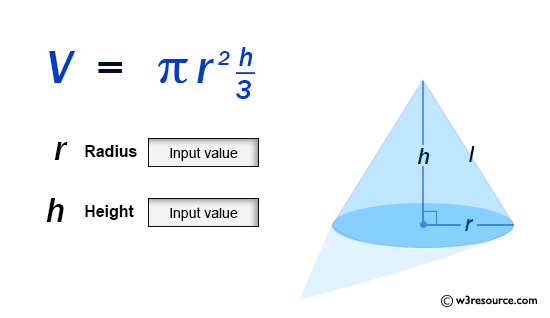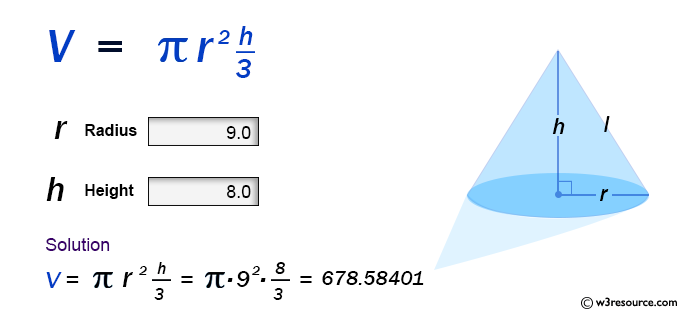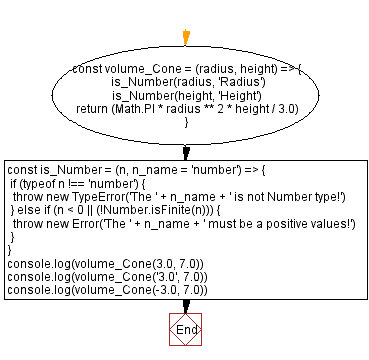# JavaScript: Volume of a Cone

## JavaScript Math: Exercise-58 with Solution

Write a JavaScript program to calculate the volume of a Cone.

From Wikipedia -
A cone is a three-dimensional geometric shape that tapers smoothly from a flat base (frequently, though not necessarily, circular) to a point called the apex or vertex.
A cone is formed by a set of line segments, half-lines, or lines connecting a common point, the apex, to all of the points on a base that is in a plane that does not contain the apex. Depending on the author, the base may be restricted to be a circle, any one-dimensional quadratic form in the plane, any closed one-dimensional figure, or any of the above plus all the enclosed points.

Sample Data:Sample Solution:

HTML Code:

``````<!DOCTYPE html>
<html>
<meta charset="utf-8">
<title>JavaScript function to Volume of a Cone</title>
<body>

</body>
</html>
```
```

JavaScript Code:

``````const volume_Cone = (radius, height) => {
is_Number(height, 'Height')
return (Math.PI * radius ** 2 * height / 3.0)
}
const is_Number = (n, n_name = 'number') => {
if (typeof n !== 'number') {
throw new TypeError('The ' + n_name + ' is not Number type!')
} else if (n < 0 || (!Number.isFinite(n))) {
throw new Error('The ' + n_name + ' must be a positive values!')
}
}
console.log(volume_Cone(3.0, 7.0))
console.log(volume_Cone('3.0', 7.0))
console.log(volume_Cone(-3.0, 7.0))
```
```

Sample Output:

```65.97344572538566
---------------------------------------------------
Uncaught TypeError: The Radius is not Number type!
at https://cdpn.io/cpe/boomboom/pen.js?key=pen.js-655e084e-84a8-8f74-b8d6-73c71300d6e2:8
------------------------------------------------------------------------------------------
Uncaught Error: The Radius must be a positive values!
```

Flowchart:Live Demo:

See the Pen javascript-math-exercise-58 by w3resource (@w3resource) on CodePen.

Improve this sample solution and post your code through Disqus

Previous: Volume of a Cube.
Next: Volume of a Pyramid

What is the difficulty level of this exercise?

Test your Programming skills with w3resource's quiz.

﻿